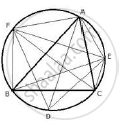Share

Bisectors of Vertex Angles A, B, and C of a Triangle Abc Intersect Its Circumcircle at the Points D, E and F Respectively. Prove that Angle Edf = 90° - 1/2 ∠A - ICSE Class 10 - Mathematics

Question

Bisectors of vertex angles A, B, and C of a triangle ABC intersect its circumcircle at the points D, E and F respectively. Prove that angle EDF  = 90° - 1/2 ∠A

SolutionJoin ED, EF and DF. Also join BF, FA, AE and EC.
∠EBF =  ∠ECF =  ∠EDF …………(i) (angles in the same segment)
In cyclic quadrilateral AFBE,
∠EBF + ∠EAF =180° ……………(ii) (sum of opposite angles)
Similarly in cyclic quadrilateral CEAF,
∠EAF + ∠ECF =180° …………. (iii)
Adding (ii) and (iii)
⇒ ∠EDF + ∠ECF + 2∠EAF = 360°
⇒ ∠EDF + ∠EDF + 2∠EAF =360°    (from (i))

⇒ 2 ∠EDF  + 2∠ EAF =  360°

⇒ ∠EDF + ∠EAF  = 180°
⇒  ∠EDF +  ∠1  + ∠BAC + ∠2 = 180°
But ∠1  = ∠3 and ∠2 = ∠4
(angles in the same segment)

∴ ∠EDF+ ∠3 + ∠BAC + ∠4  = 180°
But  ∠4 =1/2 ∠C, ∠3 = 1/2  ∠B
∴ ∠EDF + 1/2  ∠B  + ∠BAC + 1/2  ∠C  = 180°

⇒ ∠ EDF + 1/2  ∠B  + 2xx1/2 ∠A + 1/2 ∠C  = 180°
⇒ ∠EDF + 1/2 ( ∠A  + ∠B  + ∠C) + 1/2 ∠A = 180
⇒ ∠EDF + 1/2 (180°) + 1/2  ∠A  = 180°
⇒ ∠EDF + 90 + 1/2  ∠A =  180°
⇒ ∠EDF =  180° - ( 90 + 1/2 ∠ A)
⇒ ∠EDF = 180° - 90° 1/2 ∠A

⇒∠EDF = 90°- 1/2 ∠A

Is there an error in this question or solution?

APPEARS IN

Solution Bisectors of Vertex Angles A, B, and C of a Triangle Abc Intersect Its Circumcircle at the Points D, E and F Respectively. Prove that Angle Edf = 90° - 1/2 ∠A Concept: Cyclic Properties.
S